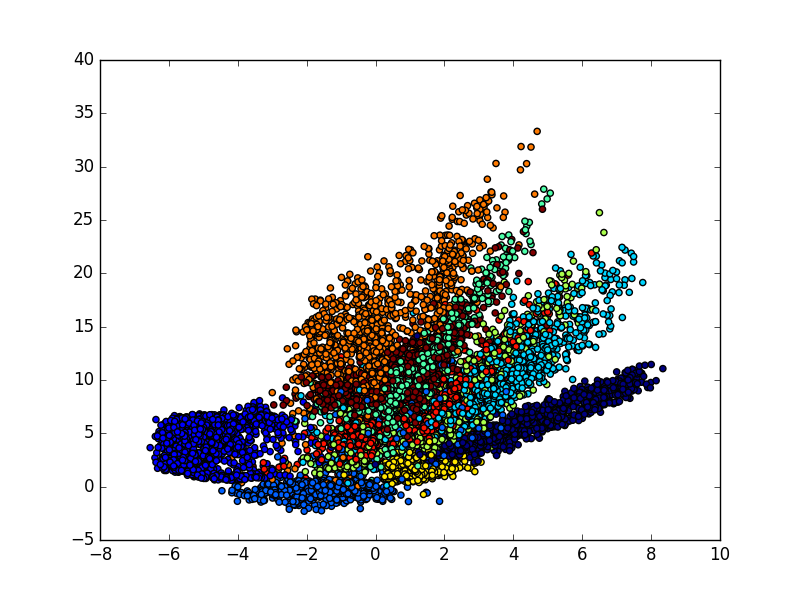# Autoencoder 自编码

## 导入模块并创建数据 ¶

import numpy as np
np.random.seed(1337)  # for reproducibility

from keras.datasets import mnist
from keras.models import Model
from keras.layers import Dense, Input
import matplotlib.pyplot as plt

# download the mnist to the path '~/.keras/datasets/' if it is the first time to be called
# X shape (60,000 28x28), y shape (10,000, )
(x_train, _), (x_test, y_test) = mnist.load_data()

# data pre-processing
x_train = x_train.astype('float32') / 255. - 0.5       # minmax_normalized
x_test = x_test.astype('float32') / 255. - 0.5         # minmax_normalized
x_train = x_train.reshape((x_train.shape, -1))
x_test = x_test.reshape((x_test.shape, -1))
print(x_train.shape)
print(x_test.shape)

"""
(60000, 784)
(10000, 784)
"""


## 建立模型 ¶

encoding_dim，要压缩成的维度。

# in order to plot in a 2D figure
encoding_dim = 2

# this is our input placeholder
input_img = Input(shape=(784,))


encoded 用4层 Dense 全联接层，激活函数用 relu，输入的维度就是前一步定义的 input_img


# encoder layers
encoded = Dense(128, activation='relu')(input_img)
encoded = Dense(64, activation='relu')(encoded)
encoded = Dense(10, activation='relu')(encoded)
encoder_output = Dense(encoding_dim)(encoded)

# decoder layers
decoded = Dense(10, activation='relu')(encoder_output)
decoded = Dense(64, activation='relu')(decoded)
decoded = Dense(128, activation='relu')(decoded)
decoded = Dense(784, activation='tanh')(decoded)

# construct the autoencoder model
autoencoder = Model(input=input_img, output=decoded)


# construct the encoder model for plotting
encoder = Model(input=input_img, output=encoder_output)


## 激活模型 ¶

# compile autoencoder


## 训练模型 ¶

# training
autoencoder.fit(x_train, x_train,
nb_epoch=20,
batch_size=256,
shuffle=True)

"""
Epoch 20/20
60000/60000 [==============================] - 7s - loss: 0.0398
"""


## 可视化结果 ¶

# plotting
encoded_imgs = encoder.predict(x_test)
plt.scatter(encoded_imgs[:, 0], encoded_imgs[:, 1], c=y_test)
plt.colorbar()
plt.show()